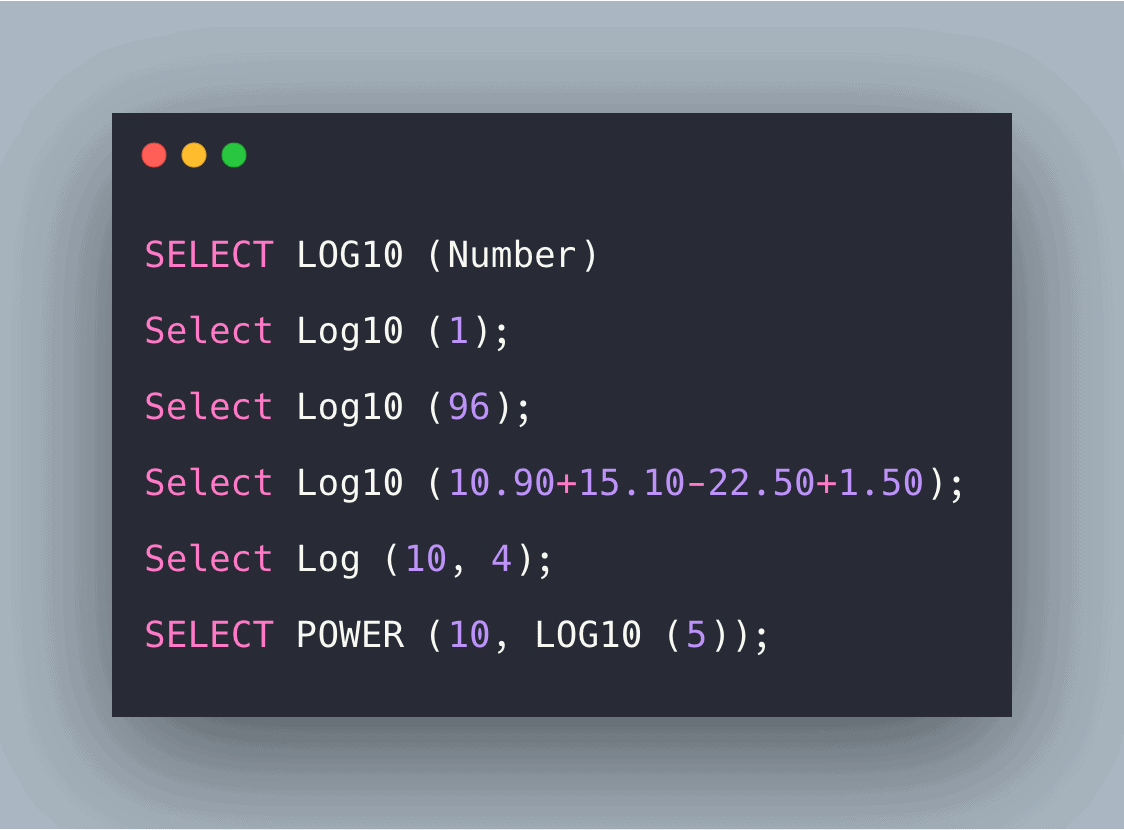# SQL Log10 Function: Complete Guide

0
3SQL log10 () function is used for returning the natural logarithm of a number to its base 10.

## SQL log10

The LOG10() is a built-in SQL function that returns the natural logarithm of a number to base 10.

### Syntax

```SELECT LOG10 (Number)
```

### Parameters

Number: The number whose logarithmic value is to be calculated. Its value should be greater than 0.

### Example

#### Query 1

```Select Log10 (1);
```

```0.0
```

#### Query 2

```Select Log10 (96);
```

#### Output

```1.9822712330395684
```

#### Query 3

```Select Log10 (10.90+15.10-22.50+1.50);
```

#### Output

```0.69897000433601886
```

#### Explanation

Here, we have directly applied the log function on the float values.

#### Query 4

```Select Log (10, 4);
```

#### Output

```0.6989700043360187
```

#### Explanation

Here 10 is the base and 4 is the number.

Similarly, it can be written as Log10 (4).

#### Query 5

```SELECT POWER (10, LOG10 (5));
```

`5`

#### Explanation

It returned the result of raising a base-10 logarithm to a specified power.

#### Query 6

```SELECT LOG10 (-1);
```

#### Output

```An invalid floating point operation occurred.
```

#### Explanation

This message was displayed because this function returns the value for only those numbers which are positiTherefore, it. It gives an error like above.

#### Query 7

```SELECT LOG10 (0);
```

#### Output

An invalid floating point operation occurred.

#### Explanation

Here, 0 was passed as an argument, so the same message was displayed which was displayed previously.

## SQL Server log10()

In SQL Server, the T-SQL LOG10() function is a mathematical function that returns the base-10 logarithm of the specified float expression.

#### Query 1

`SELECT LOG10(10) Result;`

`1`